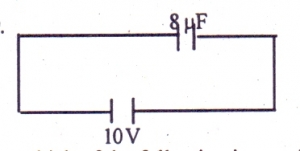# Physics 2017 Past Questions | JAMB

Study the following Physics past questions and answers for JAMBWAEC  NECO and Post-JAMB. Get prepared with official past questions and answers for upcoming examinations.

21.The net capacitance in the circuit above is
• A. 80µF
• B. 6.0µF
• C. 4.0µF
• D. 2.0µF

22. The instrument used to measure the pressure of a gas is

• A. barometer
• B. thermometer
• C. hygrometer
• D. manometer

23. A radio station broadcast at a frequency of 600KHZ. If the speed of light in air is 3 × 108ms$$^{−1}$$. Calculate the wavelength of the radio wave

• A. 2.20 × 10$$^3$$m
• B. 5.0 × 10$$^2$$m
• C. 5.0 × 10$$^5$$m
• D. 11.8 × 10$$^{11}$$m

24. Which of the following is a scalar quantity?

• A. Tension
• B. Weight
• C. Impulse
• D. Mass

25. Determine the focal length of a thin converging lens if the power is 5.0 dioptres

• A. 0.1 m
• B. 0.2 m
• C. 2.0 m
• D. 2.5 m

26.  A string of length 5cm is extended by 0.04m when a load of 0.8kg is suspended at the end. How far will it extend if a force of 16N is applied? [g = 10ms2]

• A. 0.04m
• B. 0.12m
• C. 0.01m
• D. 0.08m

27. How can energy loss be minimized through Eddy-current?

• A. By using high resistance wire
• B. By using insulated soft iron wires
• C. By using low resistance wires
• D. Bu using turns of wires

28.From the diagram above, calculate the energy stored in the capacitor
• A. 4.0 x 102J
• B. 4.0 x 104J
• C. 8.0 x 104J
• D. 8.0 x 102J

29. A constant force of 5N acts for a 5 seconds on a mass of 5kg initially at rest. Calculate the final momentum

• A. 125kgms−1
• B. 25kgms−1
• C. 15kgms−1
• D. 0kgms−1

30.

I. Refraction

II. Interference

III. Diffraction

Which of the above properties are common to all waves?

• A. II and III only
• B. I, II and III
• C. I and II only
• D. I and III only

Scroll to Top